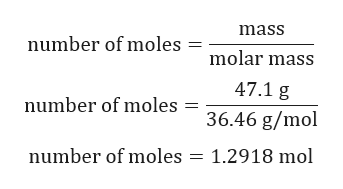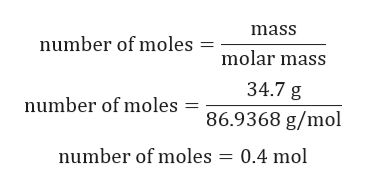# Chlorine gas can be prepared in the laboratory by the reaction of hydrochloric acid with manganese(IV) oxide. 4HCl(aq)+MnO2(s)⟶MnCl2(aq)+2H2O(l)+Cl2(g) A sample of 34.7 g MnO2 is added to a solution containing 47.1 g HCl.What is the limiting reactant?MnO2HClWhat is the theoretical yield of Cl2?theoretical yield: g Cl2If the yield of the reaction is 86.9%, what is the actual yield of chlorine?actual yield: g Cl2

Question
282 views

Chlorine gas can be prepared in the laboratory by the reaction of hydrochloric acid with manganese(IV) oxide.

4HCl(aq)+MnO2(s)⟶MnCl2(aq)+2H2O(l)+Cl2(g)

A sample of 34.7 g MnO2 is added to a solution containing 47.1 g HCl.

What is the limiting reactant?
MnO2
HCl
What is the theoretical yield of Cl2?
theoretical yield:

g Cl2
If the yield of the reaction is 86.9%, what is the actual yield of chlorine?
actual yield:

g Cl2
check_circle

Step 1

Given:

4HCl(aq)+MnO2(s)⟶MnCl2(aq)+2H2O(l)+Cl2(g)

Step 2

Calculation for moles of HCl:help_outlineImage Transcriptionclosemass number of moles molar mass 47.1 g number of moles 36.46 g/mol number of moles 1.2918 mol fullscreen
Step 3

Calculation for moles of...help_outlineImage Transcriptionclosemass number of moles molar mass 34.7 g number of moles = 86.9368 g/mol number of moles 0.4 mol fullscreen

### Want to see the full answer?

See Solution

#### Want to see this answer and more?

Solutions are written by subject experts who are available 24/7. Questions are typically answered within 1 hour.*

See Solution
*Response times may vary by subject and question.
Tagged in

### General Chemistry